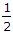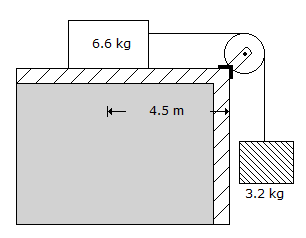# Civil Engineering - Applied Mechanics

### Exercise :: Applied Mechanics - Section 2

46.

Ball A of mass 250 g moving on a smooth horizontal table with a velocity of 10 m/s hits an identical stationary ball B on the table. If the impact is perfectly elastic, the velocity of the ball B just after impact would be

 A. zero B. 5 m/sec C. 10 m/sec D. none of these.

Explanation:

No answer description available for this question. Let us discuss.

47.

Equation of motion of a point in a straight line, is

 A. v = u + ft B. S = ut +ft2 C. 2fS = v2 - u2 D. all the above.

Explanation:

No answer description available for this question. Let us discuss.

48.

For the system of the loads shown in below figure, the time required for the 6.6 kg load to fall on the edge, isA. 1 sec. B. 2 sec. C. 3 sec. D. 4 sec. E. 5 sec.

Explanation:

No answer description available for this question. Let us discuss.

49.

One end of a light string 4 m in length is fixed to a point on a smooth wall and the other end fastened to a point on the surface of a smooth sphere of diameter 2.25 m and of weight 100 kg. The tension in the string is

 A. 17.5 kg B. 19.5 kg C. 22.5 kg D. 25 kg.

Explanation:

No answer description available for this question. Let us discuss.

50.

The inherent property of a body which offers reluctance to change its state of rest or uniform motion, is

 A. weight B. mass C. interia D. momentum.